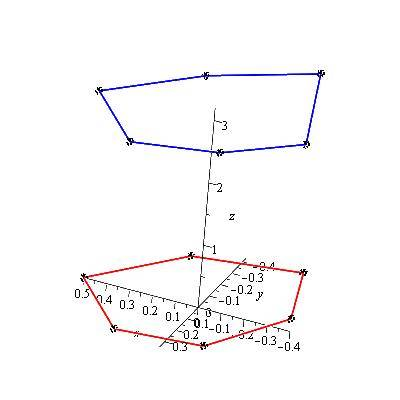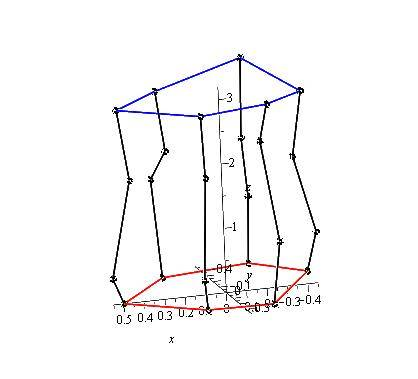# Finding volume with polar coordinate.

isdouble
The question I have is a bit strange.
I do not have ANY formulas or equations given.
I was only given bunch of points with r, theta, and z. Z being the depth. R being radius and Theta being the angle.
I was wondering if there is a way to find a rough estimate volume of the following.
R Theta Z
0.5 0 0
0.4 60 0.1
0.3 120 0
0.4 180 0.2
0.5 240 0
0.4 300 0
0.5 0 3
0.4 60 3.1
0.3 120 3.1
0.4 180 3
0.5 240 3.2
0.4 300 2.9

Of course I tried using triple integral method but was immediately out the window since there is no equations to input. Any advice would be great. Thank you.
Oh BTW, this is a irregular shape so I cannot link it to any common shapes either..

Homework Helper
Gold Member
It looks like you have 6 points around the bottom and top. If you look straight down at these points you see an irregular hexagon shape. Are the vertical sides assumed to be flat between the given points like a hexagonal cylinder or are the sides curved and just pass through the six points of the hexagon shape? I'm thinking you could take the area of the hexagon projection times the average height maybe? What else do you know about the shape?

isdouble
I got nothing. My prof just gave me these points and told me to find an estimated volume by using Excel VBA but I can't even use the VBA if I cant even solve the problem on paper. She told me there is no right or wrong answer so am I suppose to just guess what it's suppose to be? I was thinking about the same as you but that would be my last choice. Thanks for your input.

Homework Helper
Gold Member
Here's a picture. The scale on the vertical axis is compressed. One could certainly imagine a cylinder like surface.isdouble
That's one part. I forgot to mention the second part. BTW thanks for the drawing. It's really helping. So for part 1, I'm going to assume that its a cylinder and use the volume formula to find it. For part B, there are more points added to the original.
R Theta Z
0.55 0 0.4
0.45 60 0.6
0.35 120 1
0.45 180 0.8
0.55 240 1
0.45 300 1.5
0.45 0 1.9
0.35 60 2.1
0.25 120 2.5
0.35 180 2
0.45 240 2
0.35 300 2
Now it changed the form from cylinder into a irregular shape.
I can kind of visualize it like a sand clock shape. (I think?)
I'm sorry but can you draw these points on top of the ones you drew previously?
That would help me visualize better.
Thank you.

Homework Helper
Gold Member
Now it changed the form from cylinder into a irregular shape.
I can kind of visualize it like a sand clock shape. (I think?)
I'm sorry but can you draw these points on top of the ones you drew previously?
That would help me visualize better.
Thank you.

This is probably pushing the boundary of how much I should do for you:Note that the lines aren't plotted in the order the points are given. You will have to sort that out.